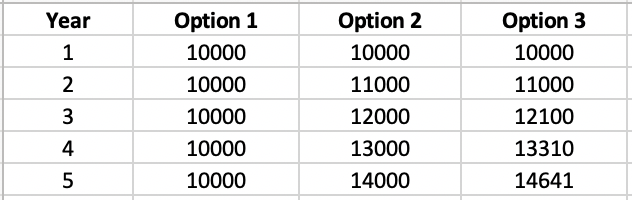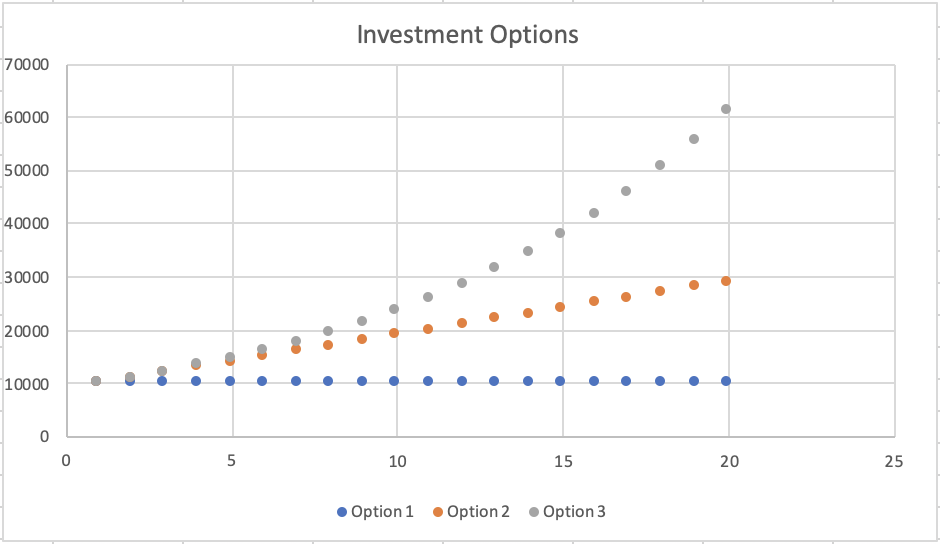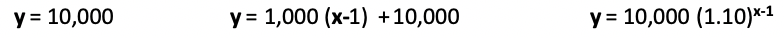# Linear Relationships – first differences

### QUESTIONWhat does it mean to use “first differences” to determine which of the 4 tables shown at right represent non-linear relationships?To find first differences, look at column 2: subtract the 1st number from the 2nd, the 2nd from the 3rd, etc.

• If these differences are all the same, then you have a linear relationship.
• If not, then the relationship is non-linear.

Why?

• Well, linear relationships have graphs that are straight lines, so their slopes are constant.
• A linear relationship is like a typical staircase, where all steps are the same height.
• When you find first differences, it’s like finding the “height” of each of the steps.

We’re making some assumptions here about the numbers in column 1:

• they are in numerical order
• their first differences are all the same: for example, 1,2,3,4,5 or 1,3,5,7,9 would be fine, but 1,2,4,5,7 or 1,3,2,5,4 would not

### BIGGER PICTURE

First differences (and second and third differences) help determine whether there is a pattern in a set of data, as well as the nature of the pattern.

For example, let’s say you invest \$1,000. Below are 3 growth options, tracked for 5 years. Which would you prefer?Which option would you prefer?

Let’s look at how your investment grows in each option:

1. In the first option, your investment grows each year by \$0. (first difference is always 0)
2. In the second option, your investment grows each year by \$1000. (first difference is always 1,000)
3. In the third option, your investment grows by \$1,000 in the 1st year, \$1,100 in the 2nd, \$1,210 in the 3rd, and so on. The annual growth increases as the years pass. (first difference keeps changing/growing)

Options 1 and 2 have constant growth (\$0 and \$1,000 annually, respectively).  Option 3 does not.

Data that has constant growth represent linear relations.

Linear relations, as the name suggests, have graphs whose points are in a straight line.

If you plotted the 3 options as a scatter plot (and extend for 20 years), it would look like this:Surprised by this graph?

Why does the 3rd option so much better than the 2nd option, when at first it seemed so similar to the 2nd option?

• Option 1 gives you an additional \$1,000 every year, which is constant growth.
• Option 2 gives you an additional 10% every year, which is exponential growth.

Here are the equations for the 3 options.(x represents the year)

###What about second differences? Let’s look at the table on the right.

• The first differences are in red.
• The second differences are in blue.

Here’s a context for this:

•column 1 could represent time
• column 2 could represent distance travelled by a falling object
• the first differences relate to the speed of the object (notice the speed keeps increasing, which makes sense for falling objects)
• the second differences would relate to the speed of the speed, or the acceleration of the object, like the acceleration due to gravity, which is constant.
• the first differences relate to the first derivative (y’)
• the second differences relate to the second derivative (y”)
##### Linear and non-linear relationships across the grades

What are the first differences in the Grade 1 growing pattern shown below?

What linear relationship does the above pattern represent?

See http://learnx.ca/growing-patterns/ for more like this, such as:

• linear and other relationships across grades 1, 4 and 9
• an interview with applied mathematician Lindi Wahl, and
• a music video from Grades 1/2 classrooms, with lyrics based on parent comments

### COMPUTATIONAL MODELLING

Click on the link below to use code to investigate first differences as well as graphs of linear relations.